• 前言
• 一、numpy是什么？
• 二、使用步骤
• 1.引入库
• 2.读入数据
• 总结

# 一、numpy是什么？

NumPy是一种非常常用的第三方模块，在学习数据分析及挖掘时经常能够用到他。接下来就阐述一些使用numpy进行的基本操作。

# 二、使用步骤

## 1.引入库

``import numpy as np ``

## 2.使用数组的基本案例

（1）创建一个长度为10，元素全为0的ndarray对象；可以使用numpy中的zeros函数

``array1=np.zeros(10)print(array1)``（2）将第3个元素改为5，第6个元素改为11

``import numpy as nparray1=np.zeros(10)array1=5array1=11print(array1)``

输出：（3）查看数组元素的数据类型

``import numpy as nparray1=np.zeros(10)array1=5array1=11print(type(array1))``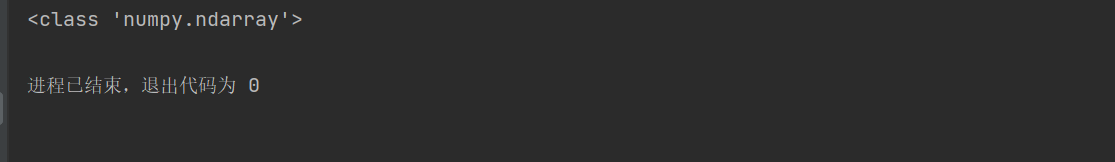3.二维数组的使用

（1）创建一个包含从10到25的16个元素的4*4的二维数组；

``import numpy as nparray1=np.arange(10,26).reshape(4,4)print(array1)``

#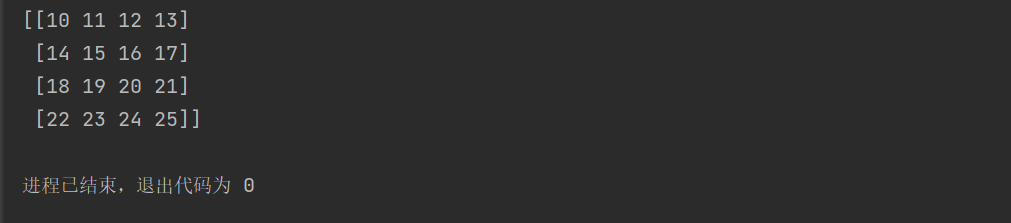​

（2）打印输出第二行、第二列的元素；

``import numpy as nparray1=np.arange(10,26).reshape(4,4)print(array1)``（3）打印输出第一行和第二行的元素；

``import numpy as nparray1=np.arange(10,26).reshape(4,4)print(array1[0:2][:])``

在调用数组时可以有多种格式，如array[0:2,:]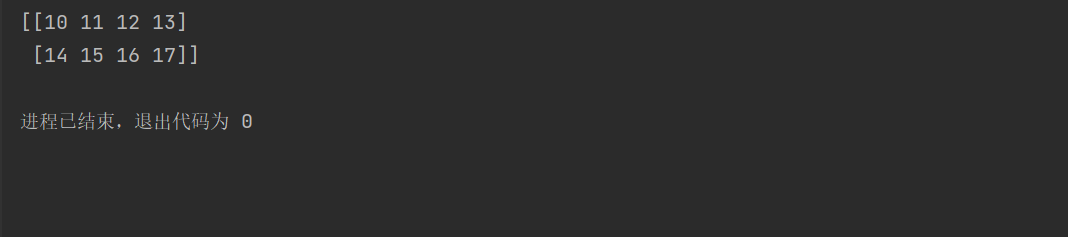（4）打印输出第一行、第三行、第一列、第三列的元素；

``import numpy as nparray1=np.arange(10,26).reshape(4,4)print(array1[0:3:2,0:3:2])``将步长设为2就可以跳过中间的一行来输出。此时如果我们使用 array1[0:3:2][0:3:2]这种写法时会发现输出的数字会自动变成同一行

``import numpy as nparray1=np.arange(10,26).reshape(4,4)print(array1[0:3:2][0:3:2])``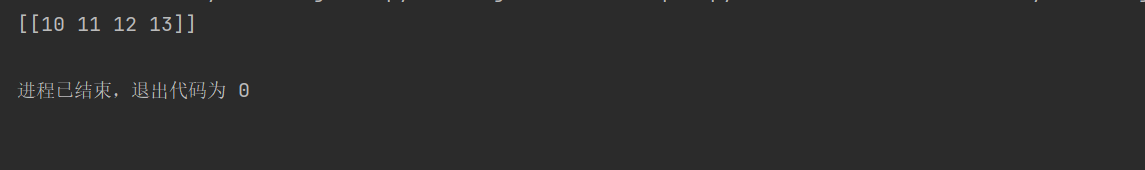4.数组的基本运算

（1）创建一个3*3*3的三维数组arr1，包含元素0~26；

``import numpy as nparr1=np.arange(0,27).reshape(3,3,3)print(arr1)``

与创建二维数组时相同的方法创建一个0到26的3*3*3数组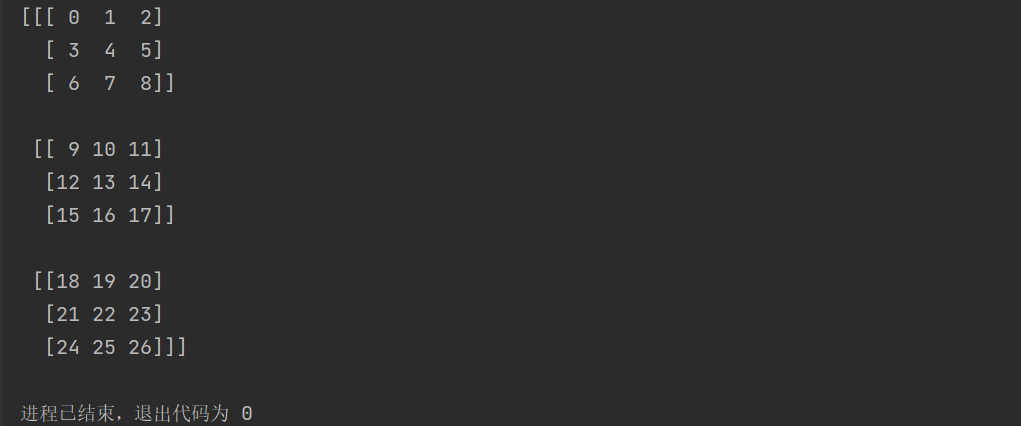（2）计算数组中各元素的平方根，得到一个新的三维数组arr2；

``import numpy as nparr1=np.arange(0,27).reshape(3,3,3)arr2=np.sqrt(arr1)print(arr2)``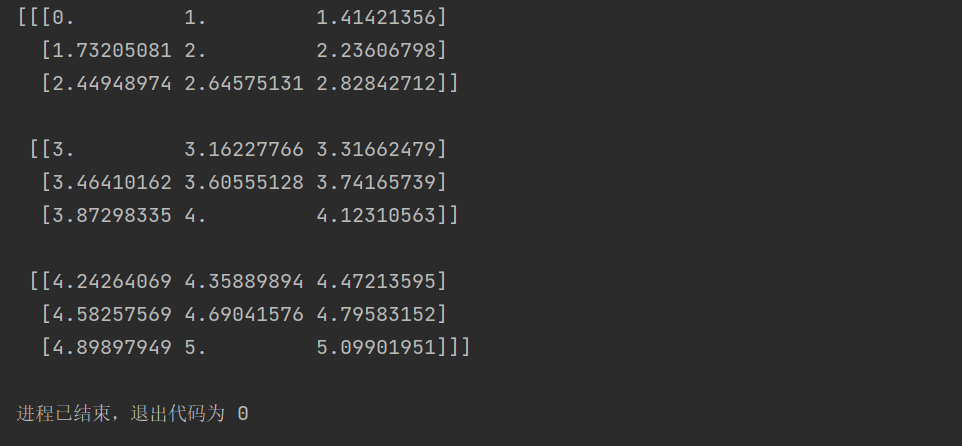``import numpy as nparr1=np.arange(-27,0).reshape(3,3,3)arr2=np.sqrt(arr1)print(arr2)``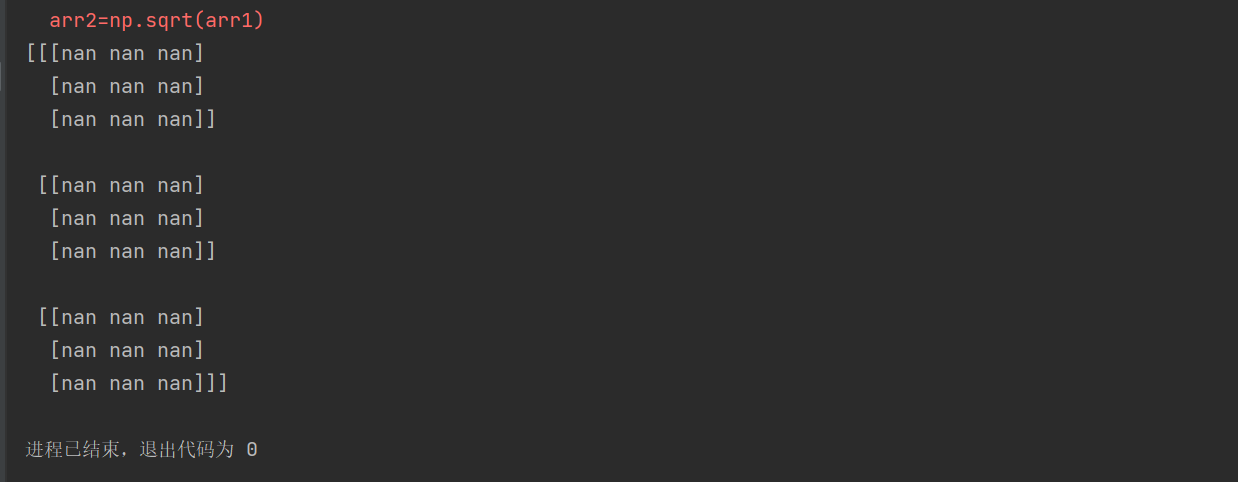（3）将arr2中的小于3的元素，改为9，其余不变；

``import numpy as nparr1=np.arange(0,27).reshape(3,3,3)arr2=np.sqrt(arr1)arr2=np.where(arr2<3,9,arr2)print(arr2)``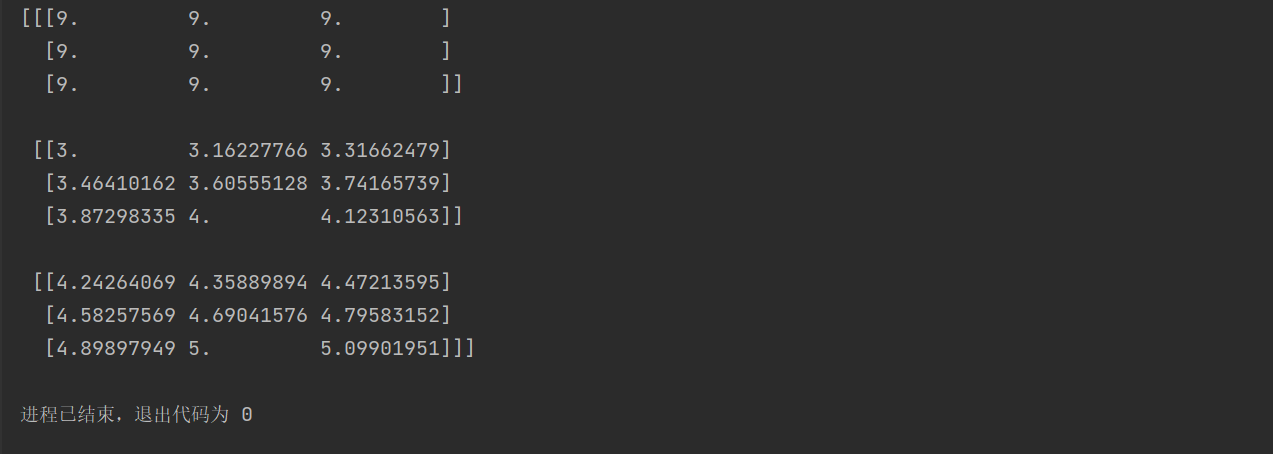（4）取出arr1中所有小于arr2中的元素，放在数组arr3中；

``import numpy as nparr1=np.arange(0,27).reshape(3,3,3)arr2=np.sqrt(arr1)arr2=np.where(arr2<3,9,arr2)arr3=arr1[arr1<arr2]print(arr3)``

在使用比较运算符时其返回的一定是一维数组